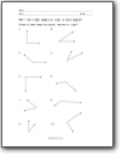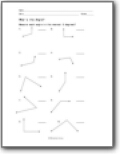# Angle Worksheets

#### What Are Angles?

Angles are used in a branch of mathematics, called geometry. This branch involves shapes and different measurements. In geometry, an angle can be defined as the figure that two rays form when they meet at a common endpoint. An angle can be represented by the symbol ∠. For instance, the angle made by the lines in the diagram can be called ∠AOB. You can use a protractor to measure an angle. The unit used is called degrees and is represented by a ° sign. There are many types of angles, and they are used in different ways in math. The types of angles include: Acute angles - Angles below 90° are called acute angles. Right angles - Angles of 90° are called right angles. Obtuse angles - Angles above 90° are called obtuse angles. Straight angles - An angle made by a straight line is of 180° and is called a straight angle. Reflex angles - An angle above 180° is called a reflex angle. Complete angles - An angle of 360° is called a complete angle because it is made by a complete circle.###### Right, Acute, or Obtuse?

Classify each angle as they are presented to you. Am I the right angle or just a cute angle?###### What's the Angle?

Measure each angle to the nearest 5 degrees?